• English：

# Decoupled Neural Interfaces using Synthetic Gradients

## Introduction

1. Forward Locking 前向锁定：在执行前向推理图中的先前节点之前，没有模块能够处理其输入数据。
2. Update Locking 更新锁定：在所有相关模块在前向图执行完毕之前，无法更新任何模块。
3. Backwards Locking 反向锁定：在所有相关模块在前馈和反向模型执行完之前，没有模块可以被更新。比如BP算法。

\begin{aligned} \frac{\partial L}{\partial \theta_{i}} &=f_{\text {Bprop }}\left(\left(h_{i}, x_{i}, y_{i}, \theta_{i}\right), \ldots\right) \frac{\partial h_{i}}{\partial \theta_{i}} \\ & \simeq \hat{f}_{\text {Bprop }}\left(h_{i}\right) \frac{\partial h_{i}}{\partial \theta_{i}} \end{aligned}

## Decoupled Neural Interfaces

Notation。我们将step为i的函数定义为fi，然后从stepi到stepj之间的函数的组成称为Fij。Layer i的损失定义为Li。

### Synthetic Gradient for Feed-Forward Networks20200218134533.png

$\theta_{i} \leftarrow \theta_{i}-\alpha \delta_{i} \frac{\partial h_{i}}{\partial \theta_{i}} ; \quad \delta_{i}=\frac{\partial L}{\partial h_{i}}$

α是学习率，对hi的求导是通过反向传播。对于sigmai的依赖意味着网络层i的更新必须在剩余部分更新后才行。即Fi+1N都已经执行了前馈和反馈阶段。因此，网络层i更新锁定在了Fi+1N。20200218135141.png

$\theta_{n} \leftarrow \theta_{n}-\alpha \hat{\delta}_{i} \frac{\partial h_{i}}{\partial \theta_{n}}, n \in\{1, \ldots, i\}$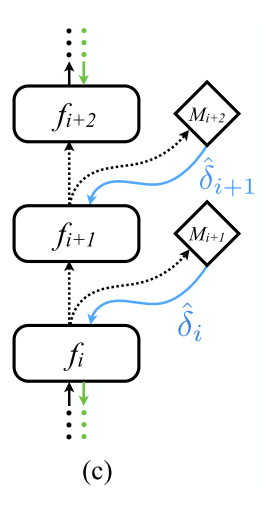20200218142500.png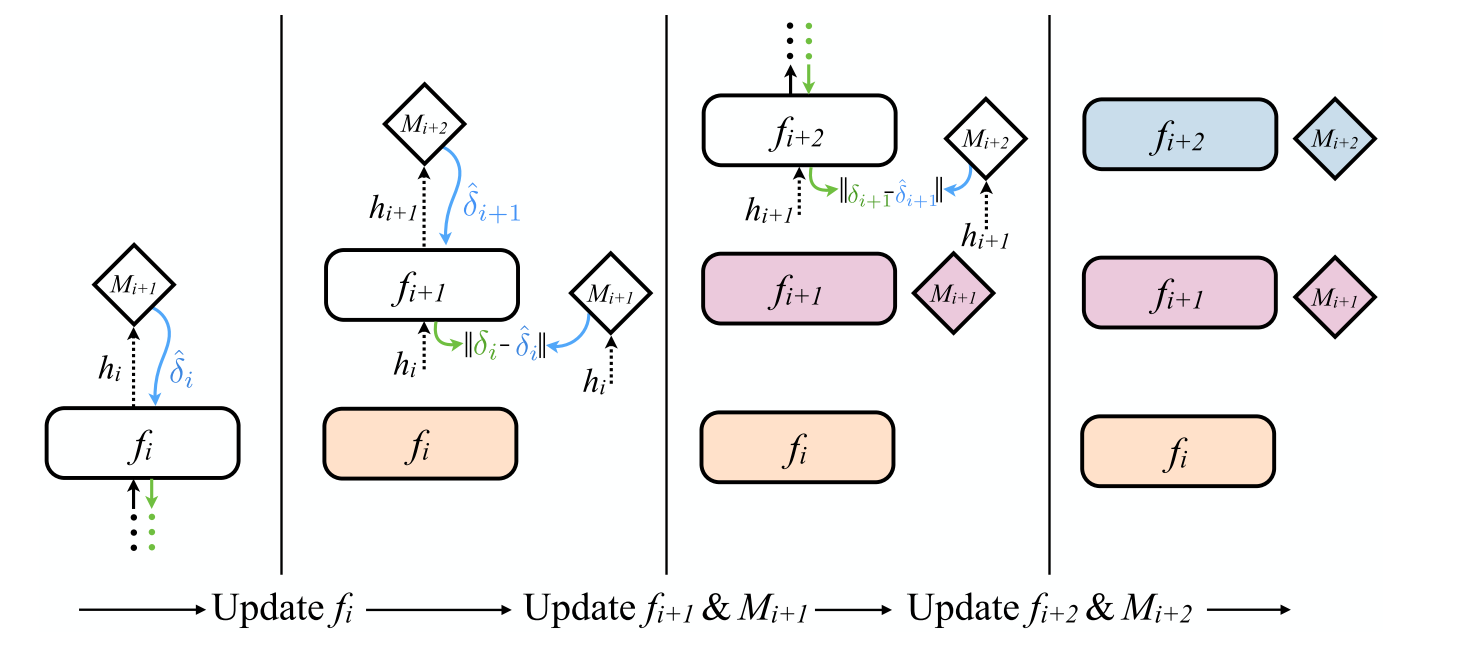20200218150932.png

$\hat{\delta}_{i} = {M_{i+1}\left(h_{i}, c\right) }$

## Experiment

### Feed-Forward Networks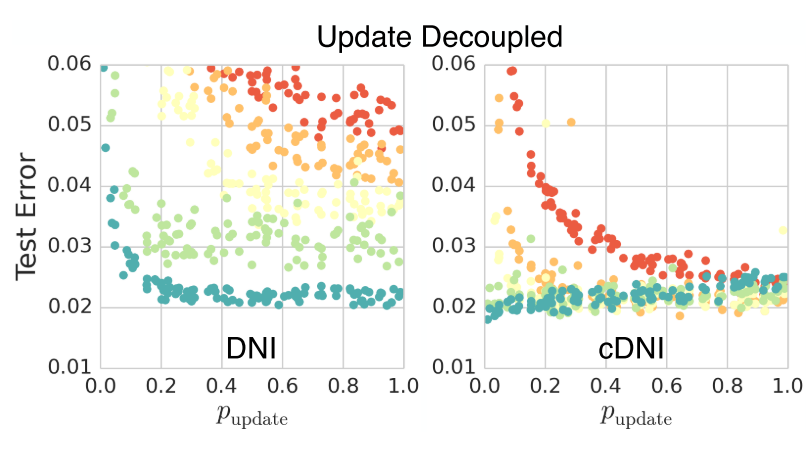20200218170640.png

### Complete Unlock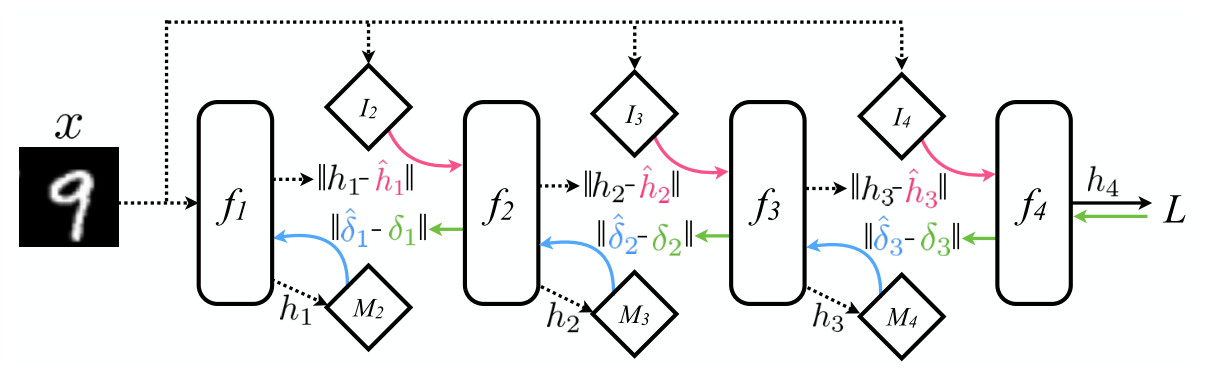20200218175611.png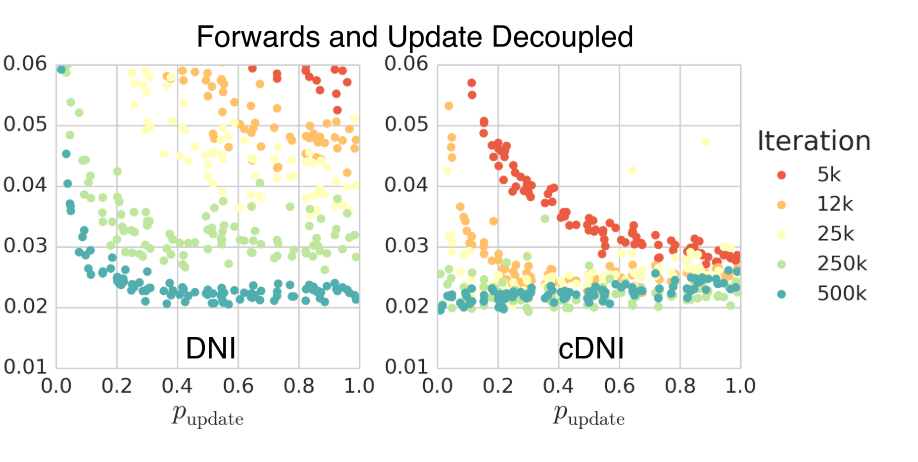20200218175713.png